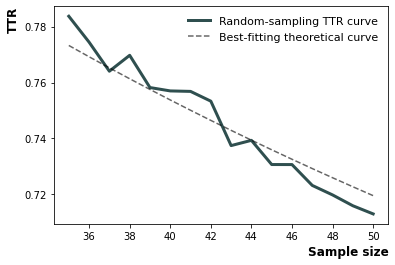# Notebook Example

:

from lexicalrichness import LexicalRichness
import lexicalrichness
lexicalrichness.__version__

:

'0.4.0'

:

# Enter your own text here if you prefer
text = """Measure of textual lexical diversity, computed as the mean length of sequential words in
a text that maintains a minimum threshold TTR score.

Iterates over words until TTR scores falls below a threshold, then increase factor
counter by 1 and start over. McCarthy and Jarvis (2010, pg. 385) recommends a factor
threshold in the range of [0.660, 0.750].
(McCarthy 2005, McCarthy and Jarvis 2010)"""

# instantiate new text object (use the tokenizer=blobber argument to use the textblob tokenizer)
lex = LexicalRichness(text)


## Attributes

:

# Get list of words
list_of_words = lex.wordlist
print(list_of_words[:10], list_of_words[-10:])

['measure', 'of', 'textual', 'lexical', 'diversity', 'computed', 'as', 'the', 'mean', 'length'] ['factor', 'threshold', 'in', 'the', 'range', 'of', 'mccarthy', 'mccarthy', 'and', 'jarvis']

:

# Return word count (w).
lex.words

:

57

:

# Return (unique) word count (t).
lex.terms

:

39


Type-token ratio (TTR; Chotlos 1944, Templin 1957):

$TTR = \frac{t}{w}$

where $$t$$ or $$t(w)$$ is the number unique terms as function of the text of length $$w$$ words.

:

# Return type-token ratio (TTR) of text.
lex.ttr

:

0.6842105263157895


Root TTR (RTTR; Guiraud 1954, 1960):

$RTTR = \frac{t}{\sqrt{w}}$
:

# Return root type-token ratio (RTTR) of text.
lex.rttr

:

5.165676192553671


Corrected TTR (RTTR; Guiraud 1954, 1960):

$CTTR = \frac{t}{\sqrt{2w}}$
:

# Return corrected type-token ratio (CTTR) of text.
lex.cttr

:

3.6526846651686067


Herdan’s C (Herdan 1960, 1964):

$C = \frac{log(t)}{log(w)}$
:

# Return Herdan's C
lex.Herdan

:

0.9061378160786574


Summer’s index (Summer 1966)

$Summer = \frac{log \log(t)}{log\log(w)}$
:

# Return Summer's index
lex.Summer

:

0.9294460323356605


Dugast’s index (Dugast 1978):

$Dugast = \frac{log(w)^2}{log(w) - log (t)}$
:

# Return Dugast's index
lex.Dugast

:

43.074336212149774


Maas’s index (Maas 1972):

$Maas = \frac{log(w) - log(t)}{log(w)^2}$
:

lex.Maas

:

0.023215679867353005


Yule’s K (Yule 1944, Tweedie and Baayen 1998):

$k = 10^4 \times \left\{\sum_{i=1}^n f(i,N) \left(\frac{i}{N}\right)^2 -\frac{1}{N} \right\}$
:

lex.yulek

:

153.8935056940597


Yule’s I (Yule 1944, Tweedie and Baayen 1998):

$I = \frac{t^2}{\sum^{n_{\text{max}}}_{i=1} i^2f(i,w) - t}$
:

lex.yulei

:

22.36764705882353


Herdan’s Vm (Herdan 1955, Tweedie and Baayen 1998):

$V_m = \sqrt{\sum^{n_{\text{max}}}_{i=1} f(i,w) \left(\frac{i}{w} \right)^2 - \frac{1}{w}}$
:

lex.herdanvm

:

0.08539428890448784


Simpson’s D (Simpson 1949, Tweedie and Baayen 1998):

$D = \sum^{n_{\text{max}}}_{i=1} f(i,w) \frac{i}{w}\frac{i-1}{w-1}$
:

lex.simpsond

:

0.015664160401002505


## Methods

### MSTTR: Mean segmental type-token ratio

• computed as average of TTR scores for segments in a text

• Split a text into segments of length segment_window. For each segment, compute the TTR. MSTTR score is the sum of these scores divided by the number of segments

• (Johnson 1944)

:

lex.msttr(
segment_window=25  # size of each segment
)

:

0.88


### MATTR: Moving average type-token ratio

• Computed using the average of TTRs over successive segments of a text

• Then take the average of all window’s TTR

• (Covington 2007, Covington and McFall 2010)

:

# Return moving average type-token ratio (MATTR).
lex.mattr(
window_size=25  # Size of each sliding window
)

:

0.8351515151515151


### MTLD: Measure of Lexical Diversity

• Computed as the mean length of sequential words in a text that maintains a minimum threshold TTR score

• Iterates over words until TTR scores falls below a threshold, then increase factor counter by 1 and start over

• (McCarthy 2005, McCarthy and Jarvis 2010)

:

lex.mtld(
# Factor threshold for MTLD.
# Algorithm skips to a new segment when TTR goes below the threshold
threshold=0.72
)

:

46.79226361031519


### voc-D

• Vocd score of lexical diversity derived from a series of TTR samplings and curve fittings

• Step 1: Take 100 random samples of 35 words from the text. Compute the mean TTR from the 100 samples

• Step 2: Repeat this procedure for samples of 36 words, 37 words, and so on, all the way to ntokens (recommended as 50 [default]). In each iteration, compute the TTR. Then get the mean TTR over the different number of tokens. So now we have an array of averaged TTR values for ntoken=35, ntoken=36,…, and so on until ntoken=50

• Step 3: Find the best-fitting curve from the empirical function of TTR to word size (ntokens). The value of D that provides the best fit is the vocd score

• Step 4: Repeat steps 1 to 3 for x number (default=3) of times before averaging D, which is the returned value

:

lex.vocd(
ntokens=50,  # Maximum number for the token/word size in the random samplings
within_sample=100,  # Number of samples
iterations=3,  # Number of times to repeat steps 1 to 3 before averaging
seed=42  # Seed for reproducibility
)

:

46.27679899103406

:

lex.vocd()

:

46.27679899103406


### voc-D plot utility

• Utility to plot empirical voc-D curve and the best fitting line

:

lex.vocd_fig(
ntokens=50,  # Maximum number for the token/word size in the random samplings
within_sample=100,  # Number of samples
seed=42,  # Seed for reproducibility
savepath="images/vocd.png",
)

:

<AxesSubplot:xlabel='Sample size', ylabel='TTR'>### HD-D

• Hypergeometric distribution diversity (HD-D) score

• (McCarthy and Jarvis 2007)

:

lex.hdd(
draws=42  # Number of random draws in the hypergeometric distribution
)

:

0.7468703323966486

[ ]: